# Package MKdescr

## Introduction

Package MKdescr includes a collection of functions that I found useful in my daily work. It contains several functions for descriptive statistical data analysis. Most of the functions were extracted from package MKmisc. Due to the growing number functions leading to an increasing number of dependencies required for MKmisc, I decided to split the package into smaller packages, where each package offers specific functionality.

library(MKdescr)

## IQR

I implemented function IQrange before the standard function IQR gained the type argument. Since 2010 (r53643, r53644) the function is identical to function IQR.

x <- rnorm(100)
IQrange(x)
##  1.261568
IQR(x)
##  1.261568

It is also possible to compute a standardized version of the IQR leading to a normal-consistent estimate of the standard deviation.

sIQR(x)
##  0.9352016
sd(x)
##  0.9408064

## Mean Absolute Deviation

The mean absolute deviation under the assumption of symmetry is a robust alternative to the sample standard deviation (see Tukey 1960).

meanAD(x)
##  0.9747385

## Huber-type Skipped Mean and SD

Huber-type skipped mean and SD are robust alternatives to the arithmetic mean and sample standard deviation (see Hampel 1985).

skippedMean(x)
##  -0.04557445
skippedSD(x)
##  0.9408064

## Five Number Summary

There is a function that computes a so-called five number summary which in contrast to function fivenum uses the first and third quartile instead of the lower and upper hinge.

fiveNS(x)
##     Minimum         25%      Median         75%     Maximum
## -1.94119839 -0.67429532 -0.04153799  0.58727246  2.07306072

## Coefficient of Variation (CV)

There are functions to compute the (classical) coefficient of variation as well as two robust variants. In case of the robust variants, the mean is replaced by the median and the SD is replaced by the (standardized) MAD and the (standardized) IQR, respectively [see Arachchige2019].

## 5% outliers
out <- rbinom(100, prob = 0.05, size = 1)
sum(out)
##  4
x <- (1-out)*rnorm(100, mean = 10, sd = 2) + out*25
CV(x)
##  0.3429777
medCV(x)
##  0.1976778
iqrCV(x)
##  0.1904704

## Signal to Noise Ratio (SNR)

There are functions to compute the (classical) signal to noise ratio as well as two robust variants. In case of the robust variants, the mean is replaced by the median and the SD is replaced by the (standardized) MAD and the (standardized) IQR, respectively.

SNR(x)
##  2.915641
medSNR(x)
##  5.058737
iqrSNR(x)
##  5.250159

## Box- and Whisker-Plot

In contrast to the standard function boxplot which uses the lower and upper hinge for defining the box and the whiskers, the function qboxplot uses the first and third quartile.

x <- rt(10, df = 3)
par(mfrow = c(1,2))
qboxplot(x, main = "1st and 3rd quartile")
boxplot(x, main = "Lower and upper hinge")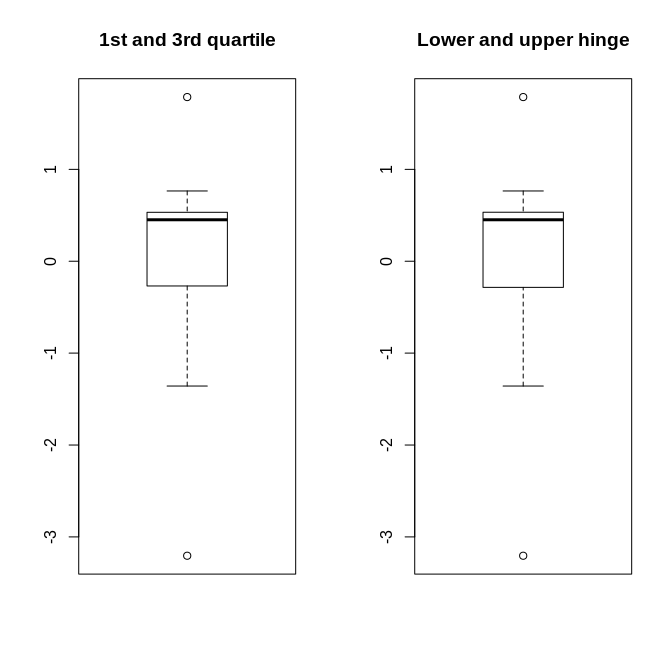The difference between the two versions often is hardly visible.

## Generalized Logarithm

The generalized logarithm, which corresponds to the shifted area hyperbolic sine, $\log(x + \sqrt{x^2 + 1}) - \log(2)$ may be useful as a variance stabilizing transformation when also negative values are present.

curve(log, from = -3, to = 5)
## Warning in log(x): NaNs wurden erzeugt
curve(glog, from = -3, to = 5, add = TRUE, col = "orange")
legend("topleft", fill = c("black", "orange"), legend = c("log", "glog"))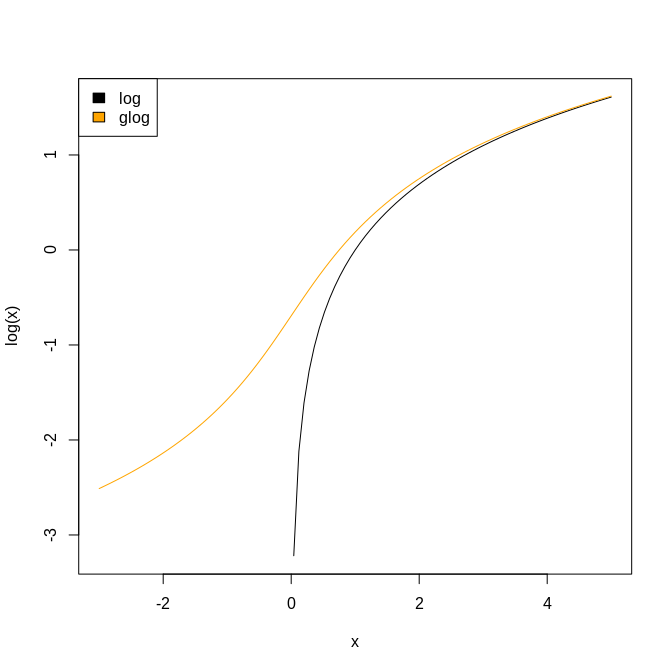As in case of function log there is also glog10 and glog2.

curve(log10(x), from = -3, to = 5)
## Warning in eval(expr, envir = ll, enclos = parent.frame()): NaNs wurden
## erzeugt
curve(glog10(x), from = -3, to = 5, add = TRUE, col = "orange")
legend("topleft", fill = c("black", "orange"), legend = c("log10", "glog10"))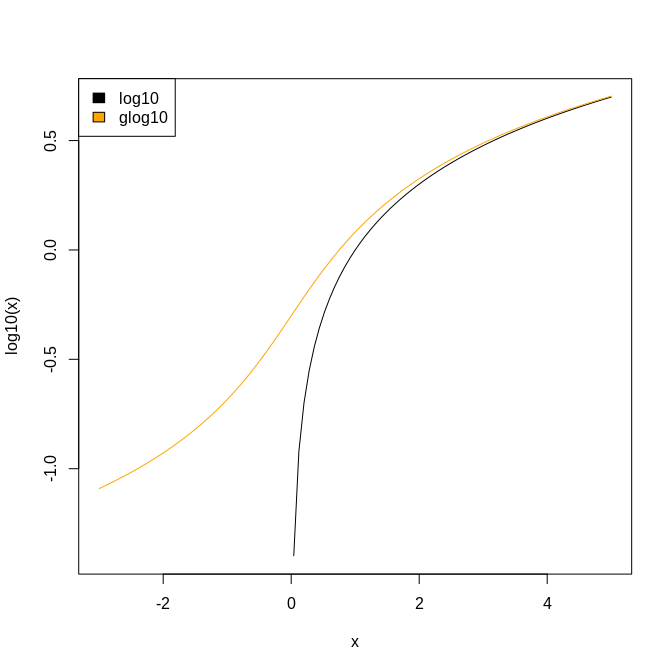There are also functions that compute the inverse of the generalized logarithm.

inv.glog(glog(10))
##  10
inv.glog(glog(10, base = 3), base = 3)
##  10
inv.glog10(glog10(10))
##  10
inv.glog2(glog2(10))
##  10

## Simulate Correlated Variables

To demonstrate Pearson correlation in my lectures, I have written this simple function to simulate correlated variables and to generate a scatter plot of the data.

res <- simCorVars(n = 500, r = 0.8)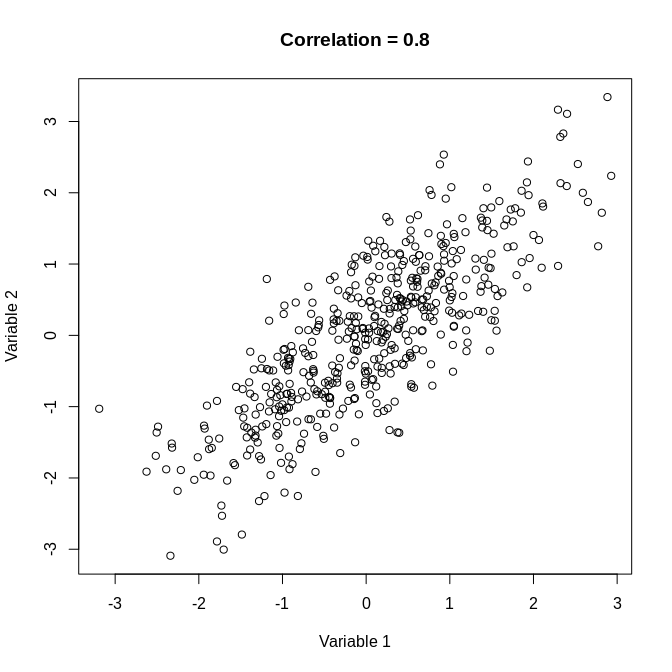cor(res$Var1, res$Var2)
##  0.8042461

## Plot TSH, fT3 and fT4 Values

The thyroid function is usually investigated by determining the values of TSH, fT3 and fT4. The function thyroid can be used to visualize the measured values as relative values with respect to the provided reference ranges.

thyroid(TSH = 1.5, fT3 = 2.5, fT4 = 14, TSHref = c(0.2, 3.0),
fT3ref = c(1.7, 4.2), fT4ref = c(7.6, 15.0))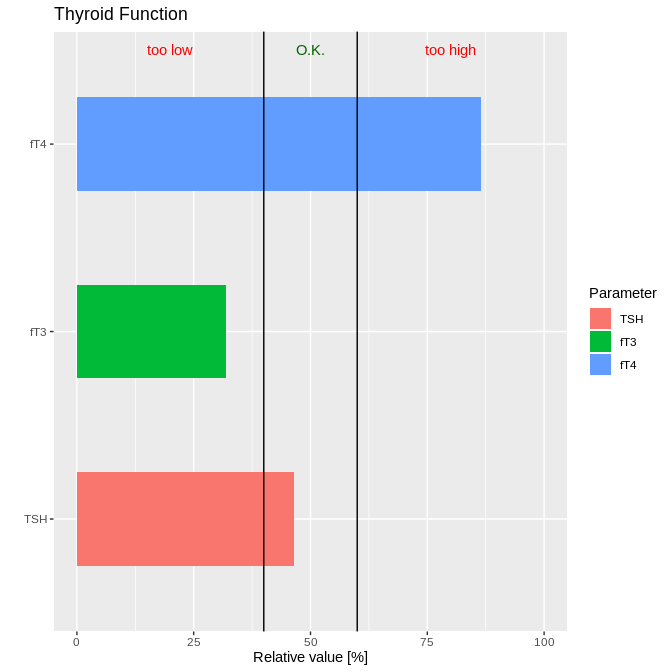## Generalized and Negative Logarithm as Transformations

We can use the generalized logarithm for transforming the axes in ggplot2 plots (Wickham 2016).

library(ggplot2)
data(mpg)
p1 <- ggplot(mpg, aes(displ, hwy)) + geom_point()
p1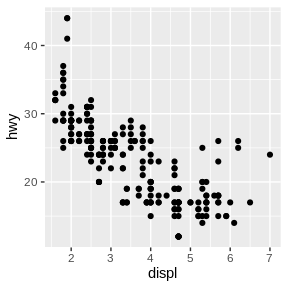p1 + scale_x_log10()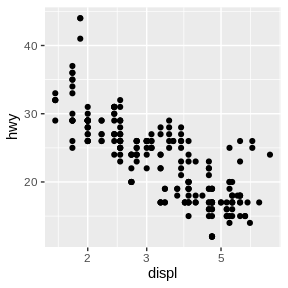p1 + scale_x_glog10()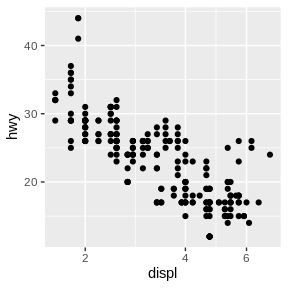p1 + scale_y_log10()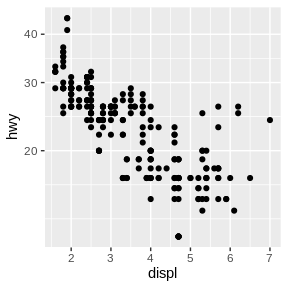p1 + scale_y_glog10()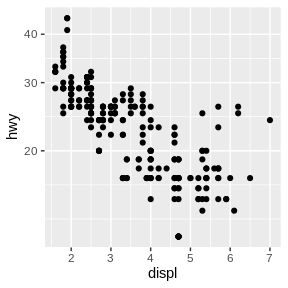The negative logrithm is for instance useful for displaying p values. The interesting values are on the top. This is for instance used in a so-called volcano plot.

x <- matrix(rnorm(1000, mean = 10), nrow = 10)
g1 <- rep("control", 10)
y1 <- matrix(rnorm(500, mean = 11.25), nrow = 10)
y2 <- matrix(rnorm(500, mean = 9.75), nrow = 10)
g2 <- rep("treatment", 10)
group <- factor(c(g1, g2))
Data <- rbind(x, cbind(y1, y2))
pvals <- apply(Data, 2, function(x, group) t.test(x ~ group)\$p.value,
group = group)
## compute log-fold change
logfc <- function(x, group){
res <- tapply(x, group, mean)
log2(res/res)
}
lfcs <- apply(Data, 2, logfc, group = group)
ps <- data.frame(pvals = pvals, logfc = lfcs)
ggplot(ps, aes(x = logfc, y = pvals)) + geom_point() +
geom_hline(yintercept = 0.05) + scale_y_neglog10() +
geom_vline(xintercept = c(-0.1, 0.1)) + xlab("log-fold change") +
ylab("-log10(p value)") + ggtitle("A Volcano Plot")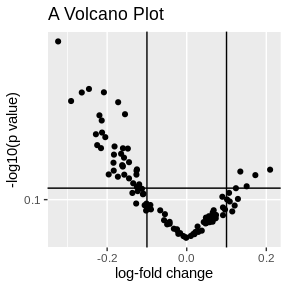## Change Data from Wide to Long

Often it’s better to have the data in a long format than in a wide format; e.g., when plotting with package ggplot2. The necessary transformation can be done with function melt.long.

library(ggplot2)
## some random data
test <- data.frame(x = rnorm(10), y = rnorm(10), z = rnorm(10))
test.long <- melt.long(test)
test.long
##           value variable
## x1   0.63325945        x
## x2  -0.66129661        x
## x3   1.00149595        x
## x4   0.35177719        x
## x5  -0.04227293        x
## x6  -0.38156478        x
## x7   1.26648474        x
## x8   0.29296165        x
## x9   0.36937718        x
## x10  0.44535828        x
## y1   0.11705993        y
## y2   1.12911810        y
## y3   0.55261465        y
## y4   0.33392152        y
## y5   0.82162372        y
## y6   1.20700278        y
## y7   0.60473536        y
## y8   0.28033459        y
## y9  -0.14697437        y
## y10 -2.30822946        y
## z1  -0.54510746        z
## z2   0.55745784        z
## z3  -0.54727297        z
## z4   0.96651399        z
## z5  -1.19991625        z
## z6   0.03666550        z
## z7  -0.54126908        z
## z8   0.91055872        z
## z9  -0.93403057        z
## z10  0.64707833        z
ggplot(test.long, aes(x = variable, y = value)) +
geom_boxplot(aes(fill = variable))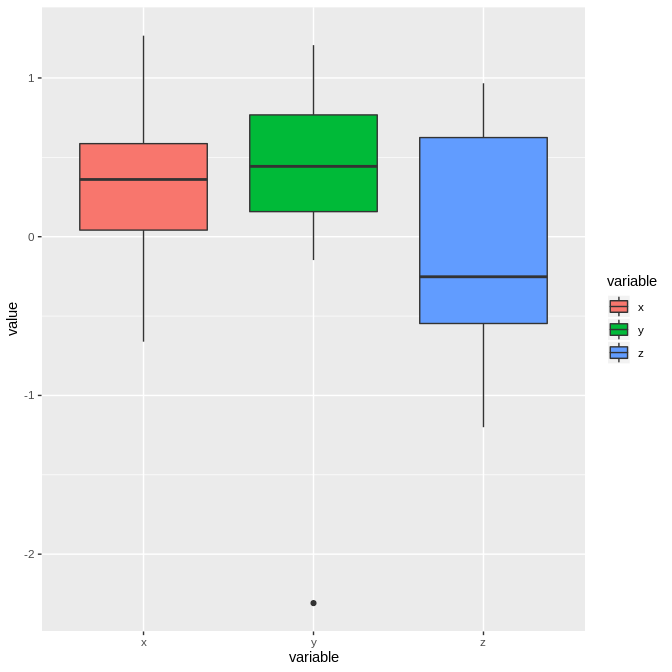## introducing an additional grouping variable
group <- factor(rep(c("a","b"), each = 5))
test.long.gr <- melt.long(test, select = 1:2, group = group)
test.long.gr
##     group       value variable
## x1      a  0.63325945        x
## x2      a -0.66129661        x
## x3      a  1.00149595        x
## x4      a  0.35177719        x
## x5      a -0.04227293        x
## x6      b -0.38156478        x
## x7      b  1.26648474        x
## x8      b  0.29296165        x
## x9      b  0.36937718        x
## x10     b  0.44535828        x
## y1      a  0.11705993        y
## y2      a  1.12911810        y
## y3      a  0.55261465        y
## y4      a  0.33392152        y
## y5      a  0.82162372        y
## y6      b  1.20700278        y
## y7      b  0.60473536        y
## y8      b  0.28033459        y
## y9      b -0.14697437        y
## y10     b -2.30822946        y
ggplot(test.long.gr, aes(x = variable, y = value, fill = group)) +
geom_boxplot()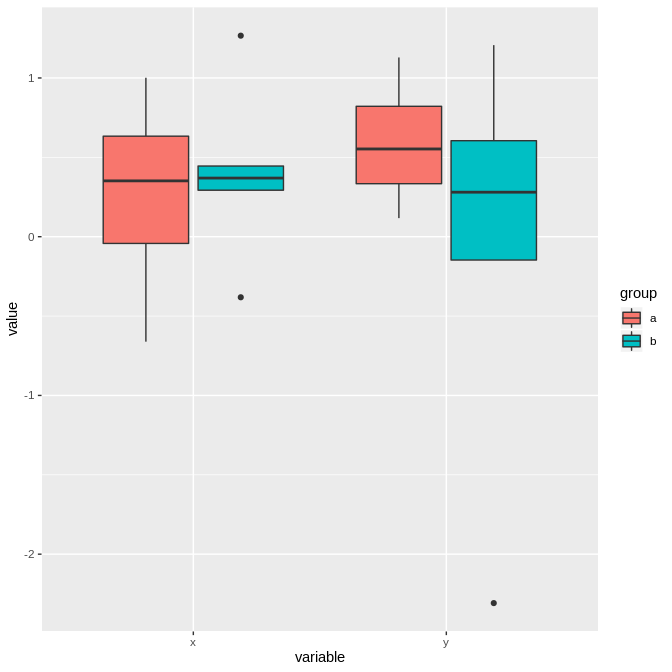## sessionInfo

sessionInfo()
## R version 3.6.1 Patched (2019-11-15 r77427)
## Platform: x86_64-pc-linux-gnu (64-bit)
## Running under: Linux Mint 19.2
##
## Matrix products: default
## BLAS:   /usr/lib/x86_64-linux-gnu/libf77blas.so.3.10.3
## LAPACK: /home/kohlm/RTOP/Rbranch/lib/libRlapack.so
##
## locale:
##   LC_CTYPE=de_DE.UTF-8       LC_NUMERIC=C
##   LC_TIME=de_DE.UTF-8        LC_COLLATE=C
##   LC_MONETARY=de_DE.UTF-8    LC_MESSAGES=de_DE.UTF-8
##   LC_PAPER=de_DE.UTF-8       LC_NAME=C
##  LC_MEASUREMENT=de_DE.UTF-8 LC_IDENTIFICATION=C
##
## attached base packages:
##  stats     graphics  grDevices utils     datasets  methods   base
##
## other attached packages:
##  ggplot2_3.2.1 MKdescr_0.5
##
## loaded via a namespace (and not attached):
##   Rcpp_1.0.2       knitr_1.25       magrittr_1.5     tidyselect_0.2.5
##   munsell_0.5.0    colorspace_1.4-1 R6_2.4.0         rlang_0.4.1
##   stringr_1.4.0    dplyr_0.8.3      tools_3.6.1      grid_3.6.1
##  gtable_0.3.0     xfun_0.10        withr_2.1.2      htmltools_0.4.0
##  assertthat_0.2.1 yaml_2.2.0       lazyeval_0.2.2   digest_0.6.22
##  tibble_2.1.3     crayon_1.3.4     purrr_0.3.3      glue_1.3.1
##  evaluate_0.14    rmarkdown_1.16   labeling_0.3     stringi_1.4.3
##  compiler_3.6.1   pillar_1.4.2     scales_1.0.0     pkgconfig_2.0.3